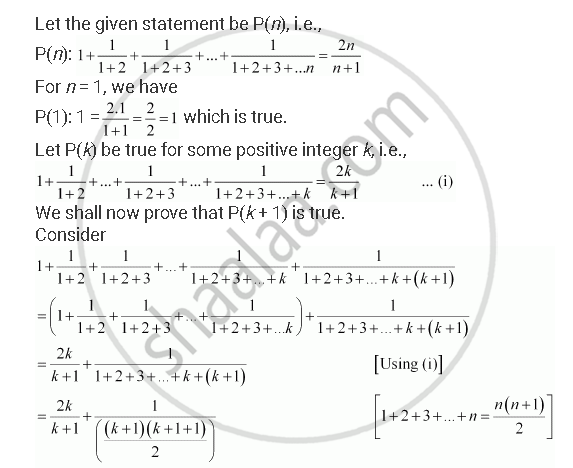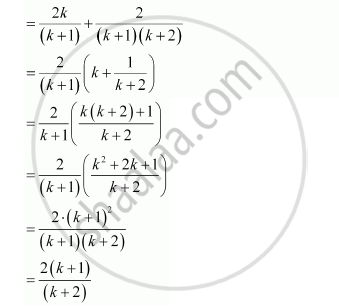CBSE (Arts) Class 11CBSE
Share

# Prove the Following by Using the Principle of Mathematical Induction for All N ∈ N: 1+ 1/((1+2)) + 1/((1+2+3)) +...+ 1/((1+2+3+...N)) = (2n)/(N +1) - CBSE (Arts) Class 11 - Mathematics

ConceptPrinciple of Mathematical Induction

#### Question

Prove the following by using the principle of mathematical induction for all n ∈ N

1+ 1/((1+2)) + 1/((1+2+3)) +...+ 1/((1+2+3+...n)) = (2n)/(n +1)

#### SolutionThus, P(k + 1) is true whenever P(k) is true.

Hence, by the principle of mathematical induction, statement P(n) is true for all natural numbers i.e., n.

Is there an error in this question or solution?

#### APPEARS IN

NCERT Solution for Mathematics Textbook for Class 11 (2018 to Current)
Chapter 4: Principle of Mathematical Induction
Q: 3 | Page no. 94

#### Video TutorialsVIEW ALL 

Solution Prove the Following by Using the Principle of Mathematical Induction for All N ∈ N: 1+ 1/((1+2)) + 1/((1+2+3)) +...+ 1/((1+2+3+...N)) = (2n)/(N +1) Concept: Principle of Mathematical Induction.
S